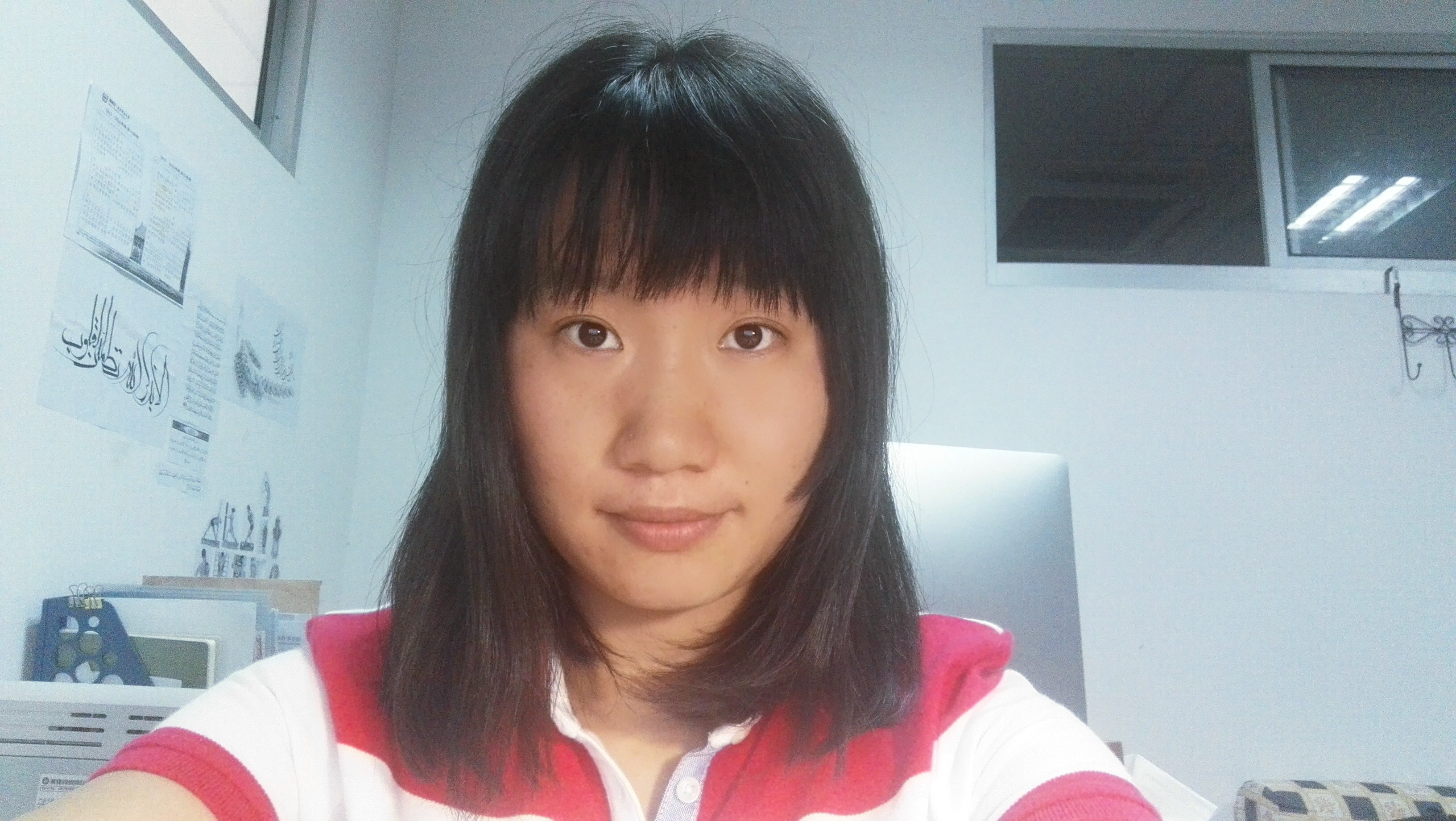Bingbing Ding Associate ProfessorResearch Interests:
Nonlinear wave equations
Bio:

Educational Background

• PhD, Applied Mathematics, Nanjing University (2015)
• BA, Information and computing science, Nanjing University of Science and Technology, (2008)

Research

Journal Articles

1. Bingbing, Ding; Huicheng, Yin,  On the blowup of classical solutions to the 3-D pressure-gradient systems. J. Differential Equations 252 (2012), no. 5,  3608–3629.
2. Ding, Bingbing; Witt, Ingo; Yin, Huicheng Blowup of classical solutions for a class of 3-D quasilinear wave equations with small initial data. Differential and Integral Equations 28 (2015), no. 9-10, 941–970.
3. Ding, Bingbing; Witt, Ingo; Yin, Huicheng, The global smooth symmetric solution to 2-D full compressible Euler system of Chaplygin gases. J. Differential Equations 258 (2015), no. 2, 445–482.
4. Ding, Bingbing; Witt, Ingo; Yin, Huicheng, On the lifespan of and the blowup mechanism for smooth solutions to a class of 2-D nonlinear wave equations with small initial data.  Quart. Appl. Math. LXXIII (2015), no. 4, 773-796.
5. Ding, Bingbing; Liu, Yingbo; Yin, Huicheng, The small data solutions of general 3D quasilinear wave equations. I, SIAM Journal on Mathematical Analysis 47, No. 6 (2015), 4192-4228.
6. Ding, Bingbing; Witt, Ingo; Yin, Huicheng, The small data solutions of general 3D quasilinear wave equations. II. J. Differential Equations, Accepted.
7. Ding, Bingbing; Witt, Ingo; Yin, Huicheng, 'Blowup time and blowup mechanism of small data solutions to general 2-D quasilinear wave equations. Communications on Pure and Applied Analysis, Accepted.

Contact

05410@njnu.edu.cn